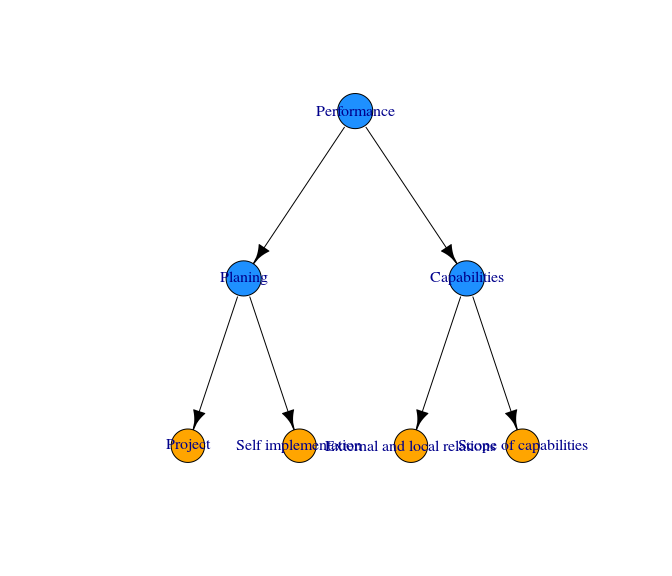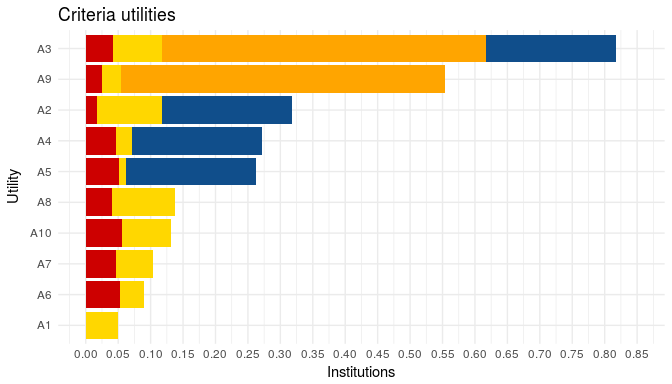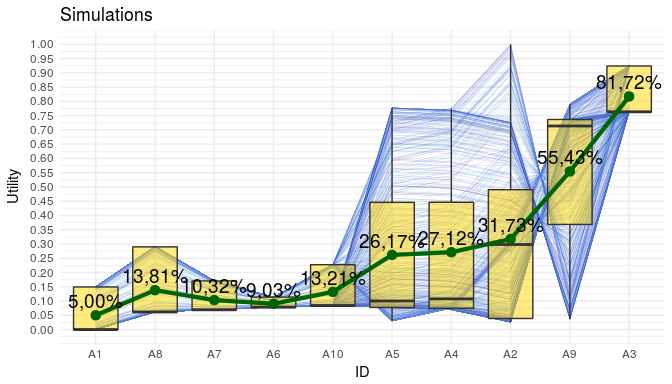# Running a MAUT Model

## Introduction

The MAUT decision models are defined with aid of utility functions $$u_1,\ldots,u_n$$ which are evaluated over indexes $$x_1,\ldots,x_n$$ and those utilities are aggregated considering additional weights $$w_1,\ldots,w_n$$, the whole final utility is given by the sum $u(x_1,\ldots,x_n) = \sum_{i=1}^n\, w_i\, u_i\ ( x_i )$ every utility $$u_i$$ can be normalized to take values only between $$0$$ and $$1$$.

A model based in Multi Attribute Utility Theory (MAUT) additionally has a decision tree that represents the relations between subsets of utilities, given an ordered and conceptual development of the decision model.

With mau you can build and test decision models based in (MAUT).

## Utility definition

The utility functions for a MAUT model could be defined in a practical format when the utilities are piecewise defined like Constant Risk Adverse Utilities (CRAU for short), satisfying the equation: $\frac{u''}{u'} = \lambda$ being $$\lambda$$ a constant. The previous equation only admits two kind of solutions, $$u(x) = a \cdot x + b$$ or $$u(x) = a \cdot e^{b \cdot x} + c$$. To determine and utility, it is only necesarry to know the parameter $$a,b,c$$. Additionally the following convention is assumed, iff $$c_i$$ is $$0$$ then the utility is linear, otherwise is an exponential function.

For piecewise CRAU, it is only necessary to define the parameters $$a,b,c$$ of the function for each part of the domain of definition. The mau package could load the utilies from an standarized text file, with the following internal structure.

mau is not only restricted to CRAU class, you can define any utility with the appropiated R script.

Header

Function name
min1 max1 a1 b1 c1
min2 max2 a2 b2 c2
min3 max3 a3 b3 c3

Function name
min1 max1 a1 b1 c1
min2 max2 a2 b2 c2
min3 max3 a3 b3 c3

Here an example of the scructure of the standarized file for utility definitions

library( mau )
file<-system.file("extdata", "utilities.txt", package = "mau" )
lines<-readLines( file )
for ( i in 1:length( lines ) ) {
cat( lines[i], '\n' )
}
## Utilities
##
## Project
##  1   2   1.5 -0.5    0
##  2   3   1.5 -0.5    0
##
## Self implementation
##  1   2   1.5 -0.5    0
##  2   3   1.5 -0.5    0
##
## External and local relations
##  1   10  1   0   0
##  0   1   0   1   0
##
## Scope of capabilities
##  6   15  1   0   0
##  0   6   1.225   -1.225  0.2824

## Main example

In the sources below is developed a complete example of a MAUT model, the package mau is employed to load utilities defined in the file utilities.txt, automatically the script with utilies is built and saved in the local working directory, after that with Eval.Utilities every function is evaluated over the columns of the index table, the names for utilities were previously standarized with Stand.String. With another file tree.csv the decision tree associated to the MAUT model is built and every weight and relative weight assigned with the Make.Decision.Tree function, in addition the whole model with utilies of every criteria is obtained with Compute.Model. The simulation of constrained weights is made with Sim.Const.Weights, the result could be employed for a sensitivy test of the decision model regarding concentrated weights variation.

1. Loading necessary packages
library( mau )
library( data.table )
library( igraph )
library( ggplot2 )
1. Index definition
index<-data.table( cod = paste( 'A', 1:10, sep = '' ),
i1 = c( 0.34, 1, 1, 1, 1, 0.2, 0.7, 0.5, 0.11, 0.8 ),
i2 = c( 0.5, 0.5, 1, 0.5, 0.3, 0.1, 0.4, 0.13, 1, 0.74 ),
i3 = c( 0.5, 1.0, 0.75, 0.25, 0.1, 0.38, 0.57, 0.97, 0.3, 0.76 ),
i4 = c( 0, 0.26, 0.67, 0.74, 0.84, 0.85, 0.74, 0.65, 0.37, 0.92 ) )
cod i1 i2 i3 i4
A1 0.34 0.50 0.50 0.00
A2 1.00 0.50 1.00 0.26
A3 1.00 1.00 0.75 0.67
A4 1.00 0.50 0.25 0.74
A5 1.00 0.30 0.10 0.84
A6 0.20 0.10 0.38 0.85
A7 0.70 0.40 0.57 0.74
A8 0.50 0.13 0.97 0.65
A9 0.11 1.00 0.30 0.37
A10 0.80 0.74 0.76 0.92
1. Loading file with utilities
file<-system.file("extdata", "utilities.txt", package = "mau" )
script<-'utilities.R'
lines<-17
skip<-2
encoding<-'utf-8'
functions<-Read.Utilities( file, script, lines, skip, encoding )
source( 'utilities.R' )

The functions data.table has the following structure with the picewise definition of CRAU’s

nom min max a b c fun
External and local relations 0 1 0.000 1.000 0.0000 external_local_relations
External and local relations 1 10 1.000 0.000 0.0000 external_local_relations
Project 1 2 1.500 -0.500 0.0000 project
Project 2 3 1.500 -0.500 0.0000 project
Scope of capabilities 0 6 1.225 -1.225 0.2824 scope_capabilities
Scope of capabilities 6 15 1.000 0.000 0.0000 scope_capabilities
Self implementation 1 2 1.500 -0.500 0.0000 self_implementation
Self implementation 2 3 1.500 -0.500 0.0000 self_implementation
1. Evaluation of utilities over every index
# Index positions
columns<-c( 2, 3, 4, 5 )

# Function names
functions<-sapply( c( 'Project',
'Self implementation',
'External and local relations',
'Scope of capabilities' ),
FUN = Stand.String )
names( functions )<-NULL

# Evaluation of utilities
utilities<-Eval.Utilities( index, columns, functions )

The utilities data.table has the following structure

cod u1 u2 u3 u4
A1 0 0 0.50 0.0000000
A2 1 0 1.00 0.0867217
A3 1 1 0.75 0.2111724
A4 1 0 0.25 0.2310170
A5 1 0 0.10 0.2586944
A6 0 0 0.38 0.2614194
A7 0 0 0.57 0.2310170
A8 0 0 0.97 0.2054301
A9 0 1 0.30 0.1215376
A10 0 0 0.76 0.2802804
1. Construction of the decision tree
file<-system.file("extdata", "tree.csv", package = "mau" )
tree.data<-Read.Tree( file, skip = 0, nrow = 8 )
tree<-Make.Decision.Tree( tree.data )
utilities<-Eval.Utilities( index, columns, functions )

plot( tree, layout = layout_as_tree )1. Computing the decision model
weights<-tree.data[ !is.na( weight ) ]$weight model<-Compute.Model( tree, utilities, weights ) 1. Bar plot for every utility xlab<-'Utility' ylab<-'Institutions' title<-'Criteria utilities' colors<-c( 'dodgerblue4', 'orange', 'gold', 'red3' ) deep<-2 bar<-Bar.Plot( model, deep, colors, title, xlab, ylab ) plot( bar )1. Global utility sensitivity under weights change. The weights are simulated employing a Dirichlet distribution. n<-800 alpha<-c( 0.2, 0.5, 0.1, 0.2 ) constraints<-list( list( c(1,2), 0.7 ), list( c(3,4), 0.3 ) ) S<-Sim.Const.Weights( n, utilities, alpha, constraints ) plot.S<-Plot.Simulation.Weight( S$simulation, title = 'Simulations',
xlab = 'ID', ylab = 'Utility' )

plot( plot.S )`